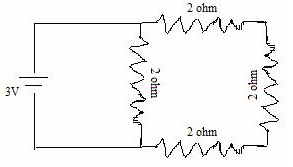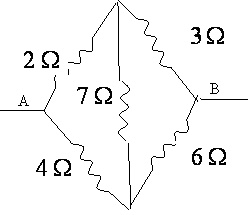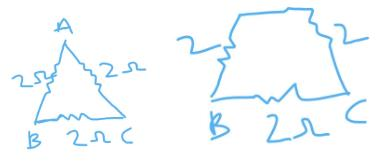Courses

# Test: Combination Of Resistors

## 5 Questions MCQ Test Physics Class 12 | Test: Combination Of Resistors

Description
This mock test of Test: Combination Of Resistors for JEE helps you for every JEE entrance exam. This contains 5 Multiple Choice Questions for JEE Test: Combination Of Resistors (mcq) to study with solutions a complete question bank. The solved questions answers in this Test: Combination Of Resistors quiz give you a good mix of easy questions and tough questions. JEE students definitely take this Test: Combination Of Resistors exercise for a better result in the exam. You can find other Test: Combination Of Resistors extra questions, long questions & short questions for JEE on EduRev as well by searching above.
QUESTION: 1

### What is current I in the circuit as shown in figure?​Solution:

Three 2Ω resistors are in series. Their total resistance =6Ω. Now it is in parallel with 2Ω resistor, so total resistance,
1/R​=1/2+1/6​=3+1/6​=4/6=2/3
R=3/2​
∴I=RV​=3/(3/2)​=3×2​/3=2A

QUESTION: 2

### Five resistances are connected as shown in fig. The effective resistance between points A and B isSolution:

In the given circuit, the arrangement of resistances is a form of Wheatstone's bridge. Hence no current will flow through the 7Ω resistor. The resistances between A and B will be a parallel combination of each other.
Hence,
1/Req​​=(1/2+3)​+(1/4+6)​
1/Req​​=(1/5)+(1/10)
∴Req​=(5×10/15)​
∴Req​=(10​/3) Ω

QUESTION: 3

### Three resistors of 4Ω, 12Ω , and 6Ω are connected in parallel. No. of 12Ω resistors required to be connected in parallel to reduce the total resistance to half of its original is

Solution:

Here 4 Ω, 12 Ω, 6 Ω when connected in parallel results in 2Ω. to reduce it to half we have to join 1\R original = 6\12 for reducing it to half we have to join 6 , 12 Ω  resistors in parallel (6\12) + (1\ 12 × 6) = 12\12 = 1 ohm . Half of its original value therefore option a is correct.

QUESTION: 4

The current in a coil of resistance 90 ohms is to be reduced by 90 percent. What value of resistance should be connected in parallel with it?

Solution:

We know that,
I=V/R​
∴​I1/I2 ​​= ​R2/ R1
​​R1​=90Ω​
Current flowing through 90Ω resistance is reduced by 90%
∴ Current ratio =10%:90%
=1:9
∴1/9​=R2​R1
​​⇒1/9​= R2​/90
​⇒R2​=90/9​=10
∴R2​=shunt=10Ω

QUESTION: 5

The equivalent resistance in a parallel resistance circuit when resistances are 2 Ω, 3 Ω and 6 Ω, and V = 6 V is

Solution:

If total resistance of wire = 6 ohm.
Then each side of an equilateral triangle has 6/3 = 2 ohm.
Diagram :If we choose BC.
Then AB and AC are in series with BC..
Hence 1/R = 1/2 + 1/4
=) 1/R = 3/4 ohm
=) R = 4/3 ohm.